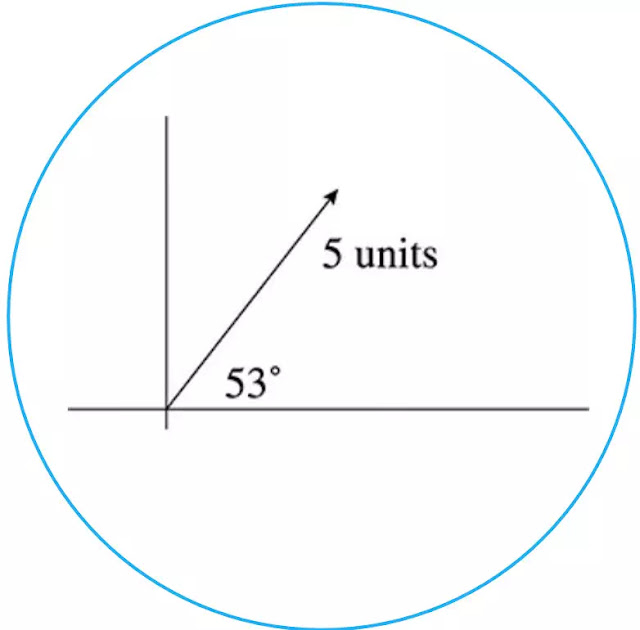### Mention the understanding of vector components?

Any vector can be broken into its x- and y-components. Here’s what we mean:

Place your finger at the tail of the vector in Figure below (that’s the end of the vectors that does not have the tip of the triangle arrow on it). Let’s say that you want to get your finger to the head of the vector without moving diagonally.

You would have to move your finger three units to the right and four units up. Therefore, the magnitude of left–right component (x component) of the vectors is "3 units" and the magnitude of up–down (y component) of the vectors is "4 units."Figure Breaking vectors into x- and y- components.

If your languages of choice are Greek and math, then you may prefer this explanation:

Given a vector V with magnitude v directed at an angle θ above the horizontal,

Vx = v cos θ

Vy = v sin θ

You may want to check to see that these formulas work by plugging in the values from our last example.

Vx = 5 cos 53° = 3 units
Vy = 5 sin 53° = 4 units

Even though the vector formulas in the box are not on the equations sheet, they are very important to memorize. You will use them in countless problems.